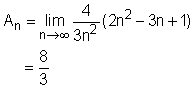Ch 6. Integrals Multimedia Engineering Math IndefiniteIntegral Area DefiniteIntegral FundamentalTheorem SubstitutionRule
 Chapter 1. Limits 2. Derivatives I 3. Derivatives II 4. Mean Value 5. Curve Sketching 6. Integrals 7. Inverse Functions 8. Integration Tech. 9. Integrate App. 10. Parametric Eqs. 11. Polar Coord. 12. Series Appendix Basic Math Units Search eBooks Dynamics Fluids Math Mechanics Statics Thermodynamics Author(s): Hengzhong Wen Chean Chin Ngo Meirong Huang Kurt Gramoll ©Kurt GramollMATHEMATICS - THEORY This section introduces a method to calculate area under a curve. Sigma Notation The Greek letter, Σ, is used to represent the sum of a series and is called sigma notation. It allows long sums (with many of terms) to be written in condensed form. In mathematics it is defined as following: If am, am+1, ... , an-1, an are real numbers and m and n are integers such that m < n, then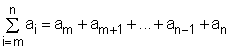Rules for Sigma Notation The following formulas are some of the basic rules fro sigma notation in which c is a constant: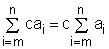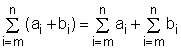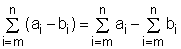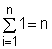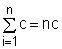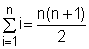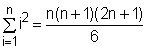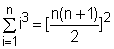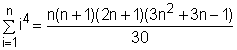The sigma notation can be used in many fields of study. besides engineering, such as economics. One such application in engineering is calculating area using finite rectangular areas. Area In high school, the formula to calculate the area of triangle, rectangle, circle and sphere was introduced. However, some areas, such as the area under the curve y = x2, can't be calculated easily with a formula. In this case, the sigma notation can help to find the area.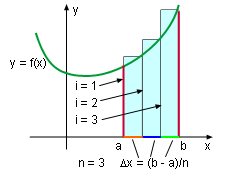Area To find the area under a curve of function y = f(x) between x = a and x = b, the interval can be equally divided into n pieces. The width of each piece is      Δx = (b-a)/n The left endpoint of these subintervals is      xi = a + iΔx in which i = 0, 1, 2, ..., n-1. These rectangles, whose height is f(xi), are placed under the curve as shown on the left diagram. The area of each rectangle is f(xi)Δx. Therefore, the area under curve y = f(x) is approximately equal to the sum area of these rectangles. It is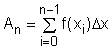The more the rectangle used, the higher the accuracy is. Thus, the area can be expressed as the limit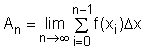Recall, previously the left edge top corner of the rectangular area was touching the curve. Now if the right side is used, the area can be expressed with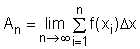These two limits both will converge to the same value as n goes to infinite.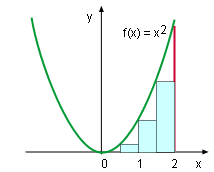The Area Under Curve y = x2 This method can be better understood by finding the area under curve y = x2 between 0 and 2. First, equally divided the interval [0, 2] into n subintervals, the width of each subinterval is 2/n. The left x coordinates of the partitions are 0/n, 2/n, 4/n, ..., 2n/n. The height of each rectangle is (xi)2. The sum of the areas of the rectangles is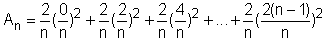which can be rewritten as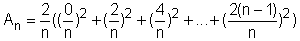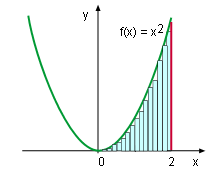Smaller Error Rearranging this expression gives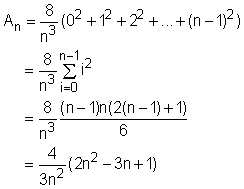Taking the limit of this expression as n approaches +∞ gives the area.